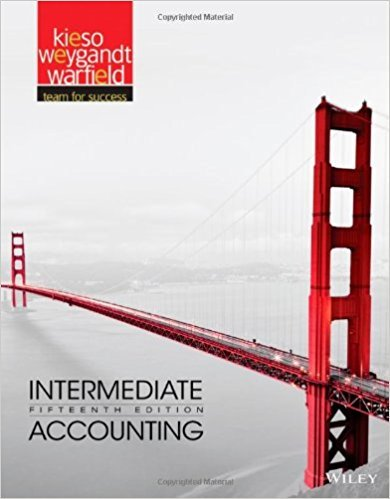×
Get Full Access to Intermediate Accounting - 15 Edition - Chapter 20 - Problem 2
Get Full Access to Intermediate Accounting - 15 Edition - Chapter 20 - Problem 2

×

# Differentiate between a defined contribution pension planISBN: 9781118147290 164

## Solution for problem 2 Chapter 20

Intermediate Accounting | 15th Edition

• Textbook Solutions
• 2901 Step-by-step solutions solved by professors and subject experts
• Get 24/7 help from StudySoup virtual teaching assistantsIntermediate Accounting | 15th Edition

4 5 1 378 Reviews
26
3
Problem 2

Differentiate between a defined contribution pension plan and a defined benefit pension plan. Explain how the employers obligation differs between the two types of plans.

Step-by-Step Solution:
Step 1 of 3

10) Use the high-low method to calculate both variable and fixed costs. ▯Slide 27 ·​ Variable Cost: (highest value-lowest value) / (highest unit - lowest unit)▯ ·​ ▯Fixed Cost: A. y = m (x) + b▯ B. y= highest or lowest value C.​ m= variable cost▯ D.​ x=number of units▯ E. b= fixed cost▯ F. highest value = variable cost (x) + fixed cost 11) Understand advantages and disadvantages of regression analysis ·​ Regression Analysis Definition: A statistical technique that separates a mixed cost into its fixed and variable components by identifying the line of best fit for the points in a data set. (A more precise approach to separating a mixed cost.)▯▯ ·​ Advantages: ▯▯ A. Statistical technique to calculate variable and fixed

Step 2 of 3

Step 3 of 3

##### ISBN: 9781118147290

This full solution covers the following key subjects: defined, plan, Pension, explain, differs. This expansive textbook survival guide covers 24 chapters, and 633 solutions. The answer to “Differentiate between a defined contribution pension plan and a defined benefit pension plan. Explain how the employers obligation differs between the two types of plans.” is broken down into a number of easy to follow steps, and 25 words. This textbook survival guide was created for the textbook: Intermediate Accounting, edition: 15. Intermediate Accounting was written by and is associated to the ISBN: 9781118147290. Since the solution to 2 from 20 chapter was answered, more than 252 students have viewed the full step-by-step answer. The full step-by-step solution to problem: 2 from chapter: 20 was answered by , our top Business solution expert on 11/23/17, 05:08AM.

Unlock Textbook Solution